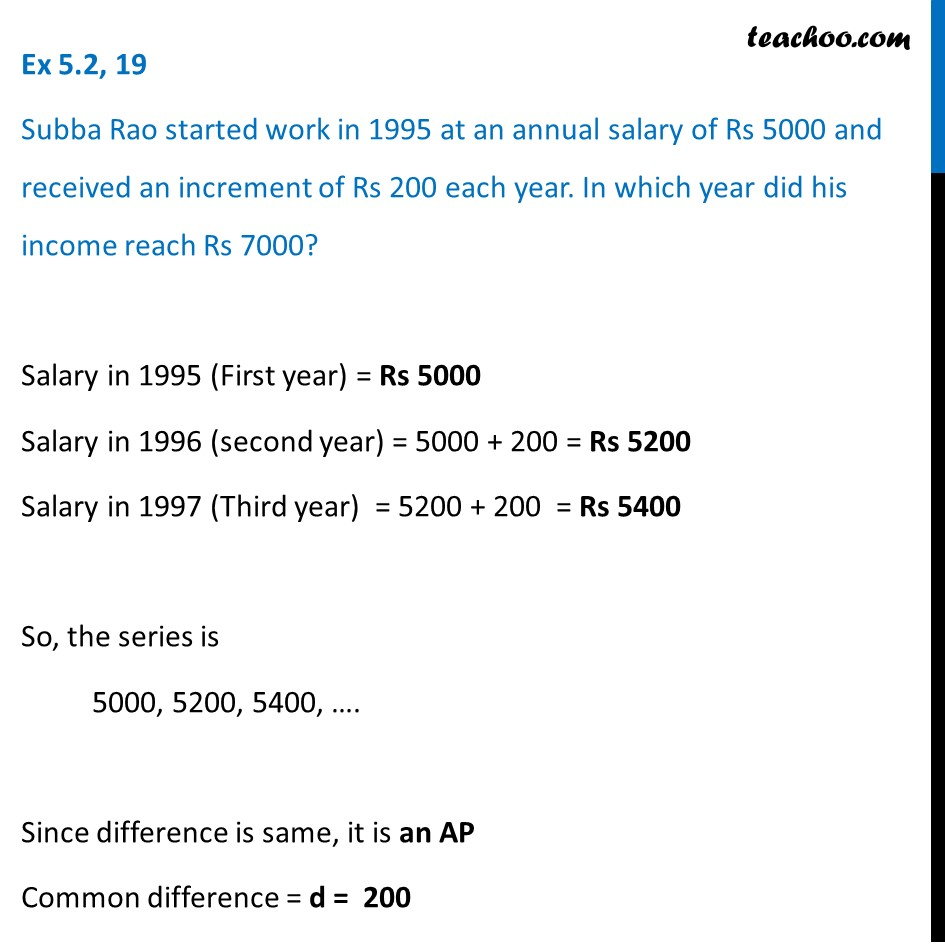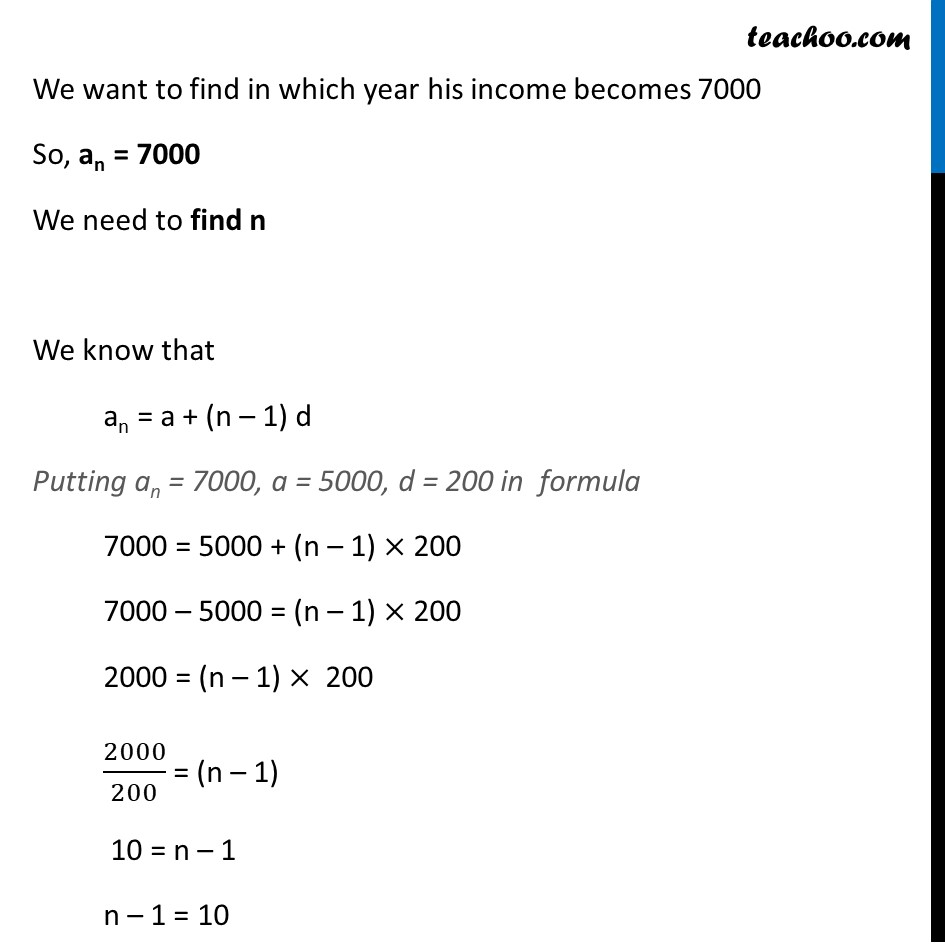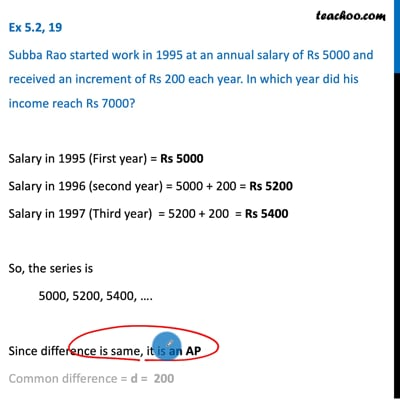Statement questions - nth term

Chapter 5 Class 10 Arithmetic Progressions (Term 2)
Concept wiseThis video is only available for Teachoo black users

### Transcript

Ex 5.2, 19 Subba Rao started work in 1995 at an annual salary of Rs 5000 and received an increment of Rs 200 each year. In which year did his income reach Rs 7000? Salary in 1995 (First year) = Rs 5000 Salary in 1996 (second year) = 5000 + 200 = Rs 5200 Salary in 1997 (Third year) = 5200 + 200 = Rs 5400 So, the series is 5000, 5200, 5400, …. Since difference is same, it is an AP Common difference = d = 200 We want to find in which year his income becomes 7000 So, an = 7000 We need to find n We know that an = a + (n – 1) d Putting an = 7000, a = 5000, d = 200 in formula 7000 = 5000 + (n – 1) × 200 7000 – 5000 = (n – 1) × 200 2000 = (n – 1) × 200 2000/200 = (n – 1) 10 = n – 1 n – 1 = 10 n = 10 + 1 = 11 Therefore, in 11th year, Subbha Rao salary has become Rs 7000 Finding 11th year 1st year = 1995 2nd year = 1996 3rd year = 1997 ….. 11th year = 1995 + 10 = 2005 ∴ In 2005, Subbha Rao’s salary has becomes Rs 7000